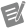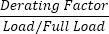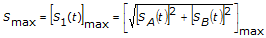From 9:00 AM - 12:00 PM CDT on Saturday, June 6th, ni.com will be undergoing system upgrades that may result in temporary service interruption.

We appreciate your patience as we improve our online experience.

# List of Features and Spectral Bands

NI InsightCM™ 3.3 Help

Edition Date: December 2018

Part Number: 374498G-01

»View Product InfoDownload Help (Windows Only)

An important part of the configuration for an asset is the lists of features and spectral bands that NI InsightCM calculates each time the asset collects data. You can review and configure the list for a given asset on the Features tab of the Asset Configuration page.

## Features

Active Power MCSA
Total input active power, in watts or kilowatts, of the motor
Apparent Power MCSA
Total input apparent power, in volt-amperes or kilovolt-amperes, of the motor
Average Temperature Thermal Imaging
The average temperature across an ROI
Crest Factor Vibration TruePeak / RMSNote  NI InsightCM Server calculates the previous features from AC-coupled waveforms. In other words, if a sensor is configured as DC-coupled, NI InsightCM Server AC couples its data for the purpose of calculating these features.
Current Vibration N/A
Delta Temperature Thermal Imaging
The difference between the maximum temperatures of two or more ROIs
Derating Factor MCSA
The value to derate the motor output based on the calculated motor voltage unbalance in compliance with NEMA MG 1-2014
Derived Peak Vibration RMS × √2
Effective Service Factor MCSAEfficiency MCSA
Motor efficiency in percentage
Envelope Total Power Vibration The total energy in the envelope spectrum.
Gap Vibration
The DC value of the signal
Humidity Vibration N/A
Line Frequency MCSA
Line frequency, in Hz, of the voltage bus
Output load, in kilowatts or horsepower, of the motor
Maximum Temperature Thermal Imaging
The highest temperature across an ROI
MCSA RMS MCSA
The RMS values of voltage or current waveforms in volts or amperes
MCSA Speed MCSA
Motor rotational speed in rotations per minute (RPM)
Minimum Temperature Thermal Imaging
The lowest temperature across an ROI
Peak-Peak Vibration The greatest positive peak minus the least negative peak
Maximum RMS for motor startup currents each cycle in percentage of the full load amperes on the motor nameplate
Motor load, in percentage of the full load on the motor nameplate
Phasor: Magnitude MCSA
Magnitude of the fundamental phasor, in volts or amperes, of voltage or current waveforms
Phasor: Phase MCSA
Phase of the fundamental phasor, in degrees, of voltage or current waveforms
Power Factor MCSA
Power factor of the motor
Reactive Power MCSA
Total input reactive power, in volt-ampere reactives or kilovolt-ampere reactives, of the motor
RMS Vibration The root mean square of the signal
Rotor Bar Sideband MCSA
Maximum magnitude, in decibels, of rotor bar sideband harmonics. The decibel reference is the fundamental component magnitude in the spectrum
Smax Vibration The maximum value of shaft vibration in two dimensions. This feature is available only for displacement sensors that are part of a pair of orthogonal probes. NI InsightCM Server also requires that each sensor in a pair have the following properties configured on the Properties tab of the Asset Configuration page. Otherwise, NI InsightCM Server logs an error value (-1, by default).
• The Pair Sensor field must specify the name of the other sensor.
• The Unit field for each sensor must match.

Smax is the result of the following equation, which complies with the ISO 79194:1996(E) standard.where S1 is the instantaneous value of the shaft displacement SA1 is the time-dependent measurement from one sensor in the pair SB1 is the time-dependent measurement from the other sensor
Speed Vibration N/A
Startup Peak Amps MCSA
Maximum instantaneous peak value, in amperes, of startup motor currents
Startup Time MCSA
Time duration, in seconds, for the motor to remain in startup state
Temperature Vibration N/A
Torque MCSA
Output torque, in Newton meters or pound-foot, of the motor
Torque Ripple MCSATotal Power in Band EMSA
The spectral energy in all frequency rangesNote  Use the Remove Spurs property for this feature to ensure that feature calculations do not include spikes.
True Peak Vibration The absolute value of the greatest positive peak or the least negative peak, whichever is greater
Unbalance MCSA
Unbalance, in percentage, of three-phase voltage buses or three-phase motor currents in compliance with NEMA MG 1-2014
Voltage Vibration N/A

## Spectral Bands

1x Magnitude Vibration The sum of the spectrum bins from 0.8x to 1.2x the speed
2x Magnitude Vibration The sum of the spectrum bins from 1.8x to 2.2x the speed
1x Phase Vibration The phase of the 1x component of the signal
2x Phase Vibration The phase of the 2x component of the signal
Asynchronous Vibration The spectral energy that is above 1x running speed and is not synchronous
EMSA Spectral Band EMSA
The spectral energy between start and stop frequenciesNote  Use the Remove Spurs property for this feature to ensure that feature calculations do not include spikes.
High Frequency Vibration The sum of the spectrum bins from 1000 Hz to the maximum frequency value
Non-synchronous Vibration The spectral energy that is not at integer multiples of running speed but is above 1x running speed
Subsynchronous Vibration The sum of the spectrum bins from 0.2x to 0.8x the speed
Synchronous Vibration The spectral energy at integer multiples of running speed
Residual Vibration A measure of the energy left in a signal after you remove the energy from all other spectral bands calculated for the sensor. For examples of this calculation, refer to the examples.

Residual spectral bands apply to a specific domain only, so the NI InsightCM web application requires you to choose the domain. For example, consider that you assign the acceleration Residual band to a sensor. The value of the band is the energy left after removing the energy from other spectral bands in the acceleration domain only. Therefore, if single integration is enabled, the acceleration Residual band factors in energy removed from a 1x Magnitude spectral band whose units are g rms, but not from a 1x Magnitude spectral band in the velocity domain whose units are ips rms.Note   You can add one Residual spectral band for each domain to a sensor. Any phase spectral bands are not part of calculating the Residual spectral band. If a sensor contains spectral bands that overlap, the algorithm removes the energy from the overlapping region only once. Therefore, the value of residual spectral bands is always greater than or equal to zero. The residual calculation accounts for the subsynchronous spectral band.

## Examples of Residual Spectral Band Values

The following table shows examples of the residual energy a device calculates given the energy in the signal and the energy in the other spectral bands the device calculates for the domain.

Energy in Signal Other Spectral Bands Residual Value
At 1x, 2x, and 3x the running speed 1x Magnitude All the energy from the 2x and 3x components of the signal
At 1x, 2x, and 3x the running speed
• 1x Magnitude
• 2x Magnitude
The energy from the 3x component of the signal
At 1x, 2x, and 3x the running speed
• 1x Magnitude
• 2x Magnitude
• A custom spectral band for 3x magnitude
Zero
At 1x, 2x, and 3x the running speed A custom spectral band from 0.8 to 3.2 orders Zero
At 1x, 2x, and 3x the running speed of 60 Hz A custom spectral band from 50 Hz to 70 Hz All the energy from the 2x and 3x components of the signal

## Related Information

Assigning Features to Sensors

Features Tab (Asset Configuration Page)

Relationship between Sensors, Measurement Types, Features, and Spectral Bands